Dr. J's Maths.com
Where the techniques of Maths
are explained in simple terms.

Functions - Transformations.
Test Yourself 1 - Solutions.

Remember when determining SHIFTS, we need to change a given equation into the form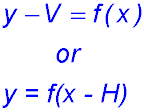When determining DILATIONS, we need to change a given equation into the formAlso remember that it is generally better to do the dilations before doing the shifts.

 Shift only 1. When y = x2 is shifted 2 units left: y = (x + 2)2 2. When y = x3 is shifted 3 units right: y = (x - 3)3 3. When y = 5x is shifted 5 units down: y + 5 = 5x or y = 5x - 5 4. When y = x - 5 is shifted 2 units up: y - 2 = x - 5 or y = x - 3 5. Shifting 3 units to the left changes y = 5x2 + 2 to y = 5(x + 3)2 + 2 6. Shifting 0.5 units up changes y = 2√x - 2.6 to y - 0.5 = 2√x - 2.6? So y = 2√x - 2.1. 7. Shifting 6 units down changes y = 5 to y = -1. 8. Shifting 2 units to the right changes x = -4 to x = -2. Dilation only 9. Dilating y = x2 vertically by a factor of 2 gives y/2 = x2 or y = 2x2. 10. Dilating y = 2x vertically by a factor of 1/3 gives 3y = 2x (after dividing y by 1/3). 11. Dilating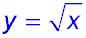horizontally by a factor of 3 gives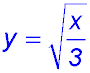. 12. Dilating y = x2 horizontally by a factor of 0.5 gives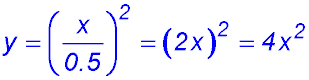13. The original parabola has x intercepts at x = -2 and x = 1 and a vertex at (-0.5, -2.25). Dilating y = (x - 1)(x + 2) horizontally by a factor of 3 (i.e. expanding or dilating it horizontally) but no shift requires dividing the x terms in BOTH bracketed terms by 3:So both the vertex and the x intercepts are dilated - i.e. multiplied by 3 - so the x intercepts change to x = -6 and x = 3 (i.e. the original values are multiplied by 3) and the new vertex is at(-1.5, -2.25). These changes are NOT the same as horizontal shifting because then all movements would be by a constant amount. 14. Dilating y = (x - 1)(x + 2) vertically by a factor of 3 gives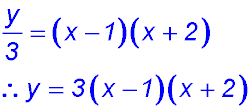Hence the x intercepts remain at x = -2 and x = 1. The x value for the vertex remains at x = -0.5 but the y value is tripled from y = -2.25 to y = -6.75. The parabola rises three times as fast as previously. Identify the shift 15. To track the change of y = 2x2 + 1 to y = 2x2 - 3 we rewrite to isolate the original equation: y = (2x2 + 1) - 4. ∴ y + 4 = (2x2 + 1) So there is a vertical shift of -4. All y values are reduced by 4. 16. To track the change of y = 4x3 to y = 4 (x + 2)3, no rewriting is necessary as it is in the relevant format. There is a horizontal shift of -2. For example the x intercept changes from x = 0 to x = -2. 17. To change y = x2 + y2 to y = (x + 1)2 + (y - 2)2 there is a horizontal shift of -1 and a vertical shift of 2. 18. To change y = sin x to y = sin (x - π) requires a horizontal shift of π units to the right. Identify the dilation 19. To transform y = 2x2 to y = 4x2, rewrite as y = 4x2 = 2(2x2).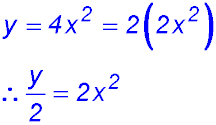Hence the effect is a vertical dilation of 2 which stretches all y values by a factor of 2 (i.e. multiplies all y values by 2). 20. To transform y = 3 cos x to y = cos x: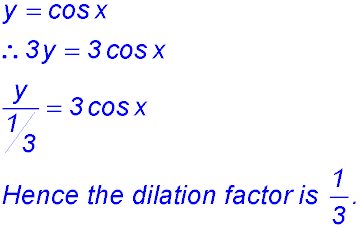The effect of this transformation is to reduce all y values by one third. So the x intercepts remain the same but the maximum values reduce from 3 to 1. 21. To transform y = 2x to y = 2x+2: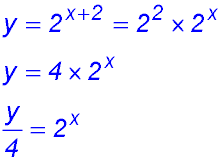The effect of the vertical dilation is to change all y values by a dilation factor of 4 (i.e. to multiply all y values by 4 - see especially x = 0).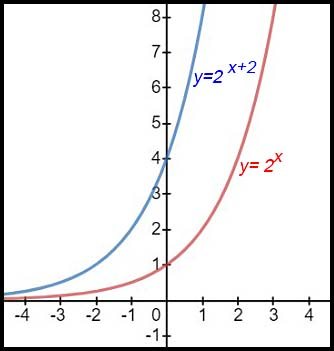22. To transform y = 2x + 4 to y = x + 2: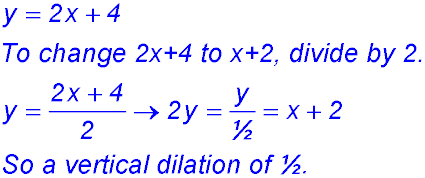The effect of the vertical dilation is to halve all y values for each x value. Hence the point (2, 8) on y = 2x + 4 becomes (2, 4) on y = x + 2.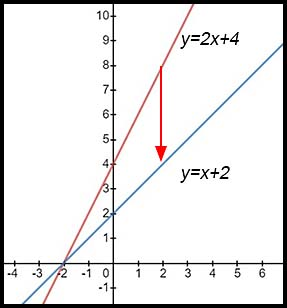Shift and dilation 23. To change x2 + y2 = 4 into (x - 3)2 + (y + 4)2 = 16: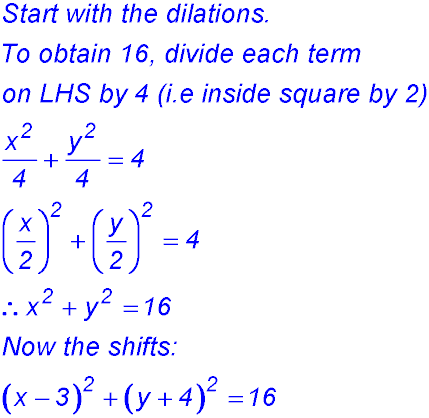horizontal shift of 3; vertical shift of -4; horizontal and vertical dilations of 2.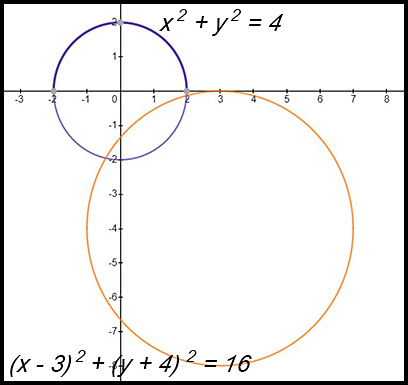The centre of the original circle is (0, 0). The centre of the transformed circle is (3, -4). The dilation transformations change the radius from 2 to 4. The shift transformations move the centre from x = 0 to x = 3 and from y = 0 to y = -4. 24. The transformation(s) to change x2 + y2 = 1 into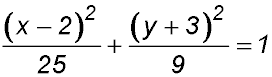require rewriting the equation as: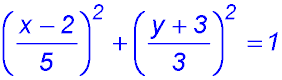horizontal shift of 2; vertical shift of -3; horizontal dilation (enlargement) of 5; vertical dilation of 3. The circle turns into a horizontal ellipse. 25. To transform y = cos x into y = 5cos(2x - π/3):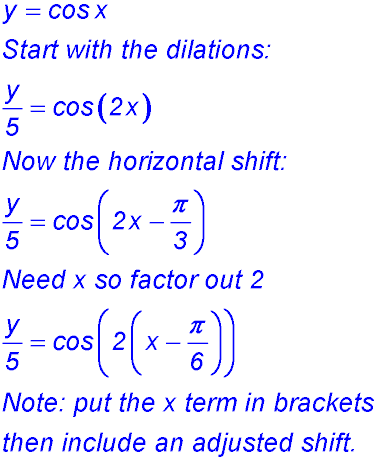So the transformations are: horizontal shift of π/6; no vertical shift; horizontal dilation factor of 0.5; vertical dilation factor of 5. 26. To transform the equation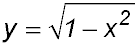to: Start with the vertical dilation: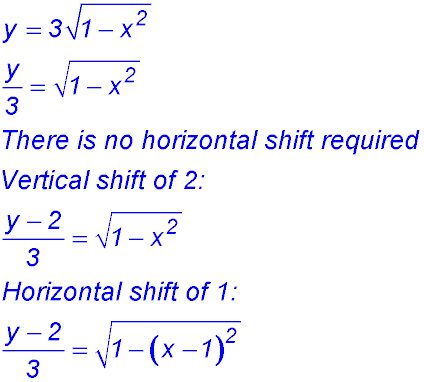So the transformations are: horizontal shift of 1; vertical shift of 2; no horizontal dilation; vertical dilation with a factor of 3.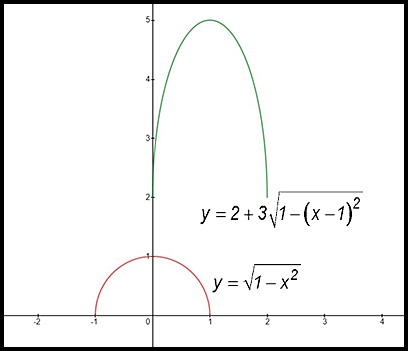The peak of the semi-circle is at (0, 1): the vertical dilation takes (0, 1) to (0, 3); the vertical shift takes (0, 3) to (0, 5); the horizontal shift takes (0, 5) to (1, 5). 27. To transform the equationto become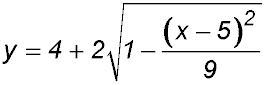: It is possible to start transforming the original equation by incorporating the vertical shift: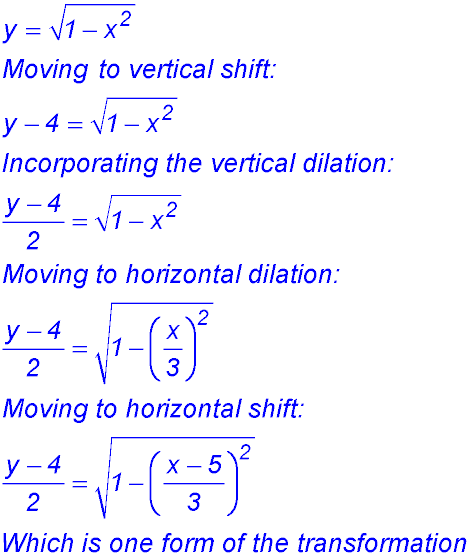Hence: the horizontal shift is 5; the vertical shift is 4; the horizontal dilation is 3; the vertical dilation is 2.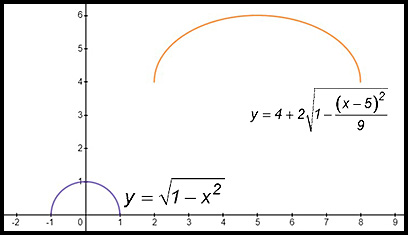Note in the above graph: the origin (0, 0) moves horizontally 5 units and vertically 4 to (5, 4); the original point (-1, 0) was 1 unit to the left of (0, 0) and the horizontal dilation of 3 results in that distance being extended to -3 units from (5, 4) - so to (2, 4). the original point (0, 1) was 1 unit above (0, 0) and the vertical dilation of 2 results in that distance being extended up 2 units from (5, 4) - so to (5, 6). 28. Starting with the equation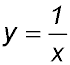what transformations change the equation to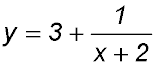?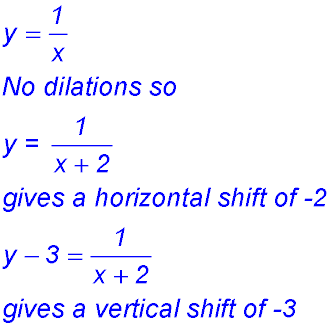Transformations are: horizontal shift of -2; vertical shift of -3; no dilations. Use the following diagram of y = f(x) = x2 to sketch new graphs in response to the information given in each of questions 29-30.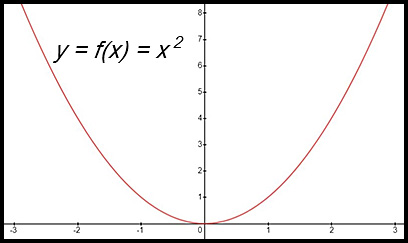29.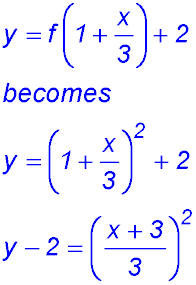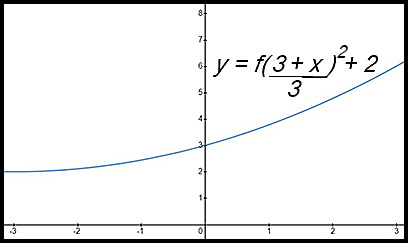Note: There is a horizontal shift of 3 to the left and a horizontal dilution (expansion) by a factor of 3 (so the curve flattens out). Hence the parabola flattens and the vertex moves to x = -3. There is a vertical shift of 2. Hence the vertex is now at (-3, 2) rather than (0, 0). At x = 0, y = 1 + 2 = 3. 30.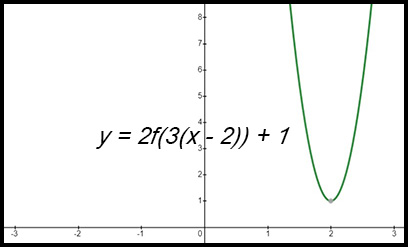Note: there is a horizontal shift of 2 to the right (so the vertex is now at x = 2) and a horizontal dilution of 1/3 (hence the curve becomes narrower). There is a vertical shift of 1 upwards (so the vertex moves to y = 1) and a vertical dilution by a factor of 2 (hence the curve climbs more quickly). Use the following diagram of y = f(x) to sketch new graphs in response to the information given in each of questions 31-34.Diagrams 31.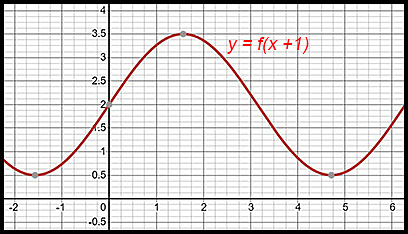Note: The horizontal shift of -1 moves the previous point at x = 1 to the left and it is now on the y axis. The minimum points at about x = -0.5 and x = 5.75 have moved left to about x = -1.5 and to about x = 4.75 . There is no vertical change. 32.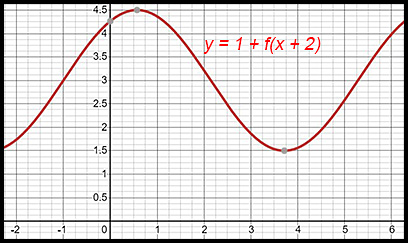Note: The previous centre of the loops was at y = 2. The vertical shift of 1 has shifted the central y value up to y = 3. The previous range was from 0.5 to 3.5 and that has changed to a range from 1.5 to 4.5. The horizontal shift of -2 moves the maximum point from about x = 2.5 to the left to x = 0.5. The next minimum at about 3.5 has therefore been shifted left to about 1.5. 33.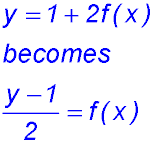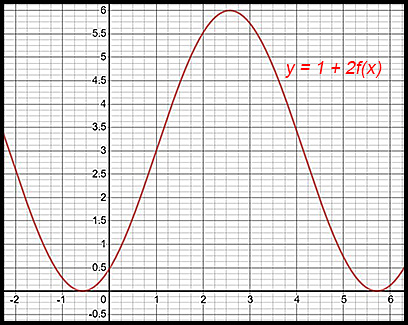Note: The previous centre of the loops was at about y = 2 with a minimum of y = 0,5 and a maximum of y = 3.5 (a difference of 3). The vertical shift of 1 moves the centre between the peaks and troughs from y = 2 to y = 3. The vertical dilation of 2 changes one minimum value to y = 0 at x = -0.5 and one maximum value to y = 6 at x = 2.5 respectively (a difference of 6 which is twice the original amplitude). There is no horizontal shift or dilation. 34.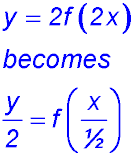Note: There is a horizontal dilation of ½ which compresses the pattern. Previously one of the lowest points occurred at x = ½ whereas now, the corresponding lowest point is at x = ¼. The distances between the two troughs was originally 6 units but is now 3 units. The distance above the y axis was originally ½ but it is now 1. The vertical dilation of a factor of 2 increases the amplitude of thepattern from 3 units to 6 units.